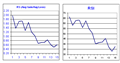# RSI (sma) code, does it look correct? - page 2181923

Johan Strandberg:

Interesting that they produce the same result (and that it's called Cutlers when using SMA). Would you know if my SMA-code looks correct? I think it's okey but would be great if I could get it confirmed.

I've followed macrooptions link and then I got unsure when looking at a regular RSI in MT4, in retrospect I now understand with the help of some previous answers that the MT4 RSI is calculated with a EMA which is why my own code based on SMA differs :)

If by "average" that they use there they mean "simple moving average", then they are describing Cuttlers' RSI there - not the Welles Wilders' original version

Fairly good description of the original RSI can be the one bellow (from here : RSI calculation - you will notice that they are describing SMMA calculation)

In Wilder's RSI, RS uses Wilder's smoothing which is a different form of exponential averaging. Wilder's smoothing = Previous MA value + (1/n periods * (Close - Previous MA))

```                  100
RSI = 100 - --------
1 + RS

RS = Average Gain / Average Loss

```

To simplify the calculation explanation, RSI has been broken down into its basic components: RS, Average Gain and Average Loss. This RSI calculation is based on 14 periods, which is the default suggested by Wilder in his book. Losses are expressed as positive values, not negative values.

The very first calculations for average gain and average loss are simple 14 period averages.

• First Average Gain = Sum of Gains over the past 14 periods / 14.
• First Average Loss = Sum of Losses over the past 14 periods / 14

The second, and subsequent, calculations are based on the prior averages and the current gain loss:

• Average Gain = [(previous Average Gain) x 13 + current Gain] / 14.
• Average Loss = [(previous Average Loss) x 13 + current Loss] / 14.Relative Strength Index (RSI) [ChartSchool]
• stockcharts.com
Developed J. Welles Wilder, the Relative Strength Index (RSI) is a momentum oscillator that measures the speed and change of price movements. RSI oscillates between zero and 100. Traditionally, and according to Wilder, RSI is considered overbought when above 70 and oversold when below 30. Signals can also be generated by looking for...